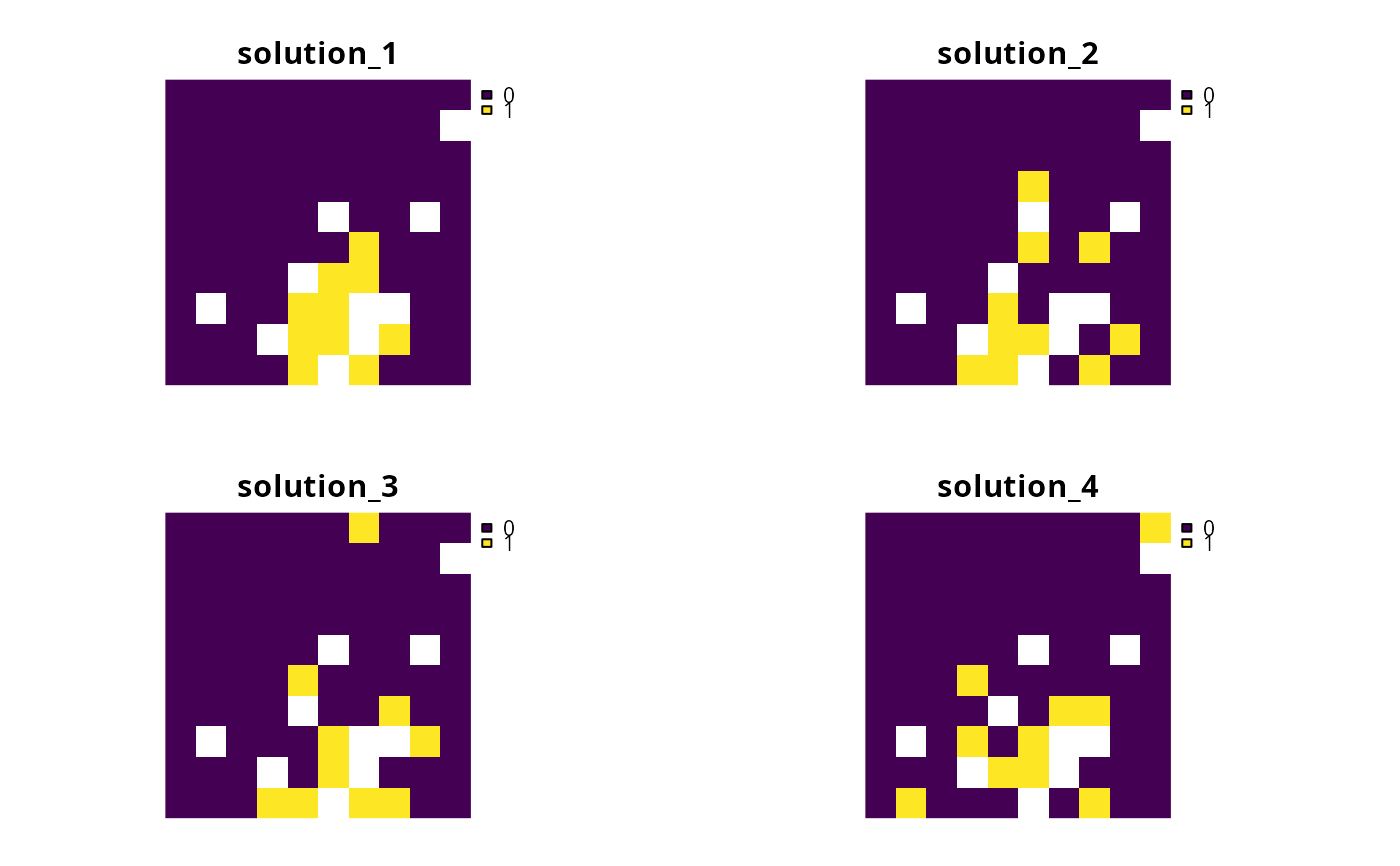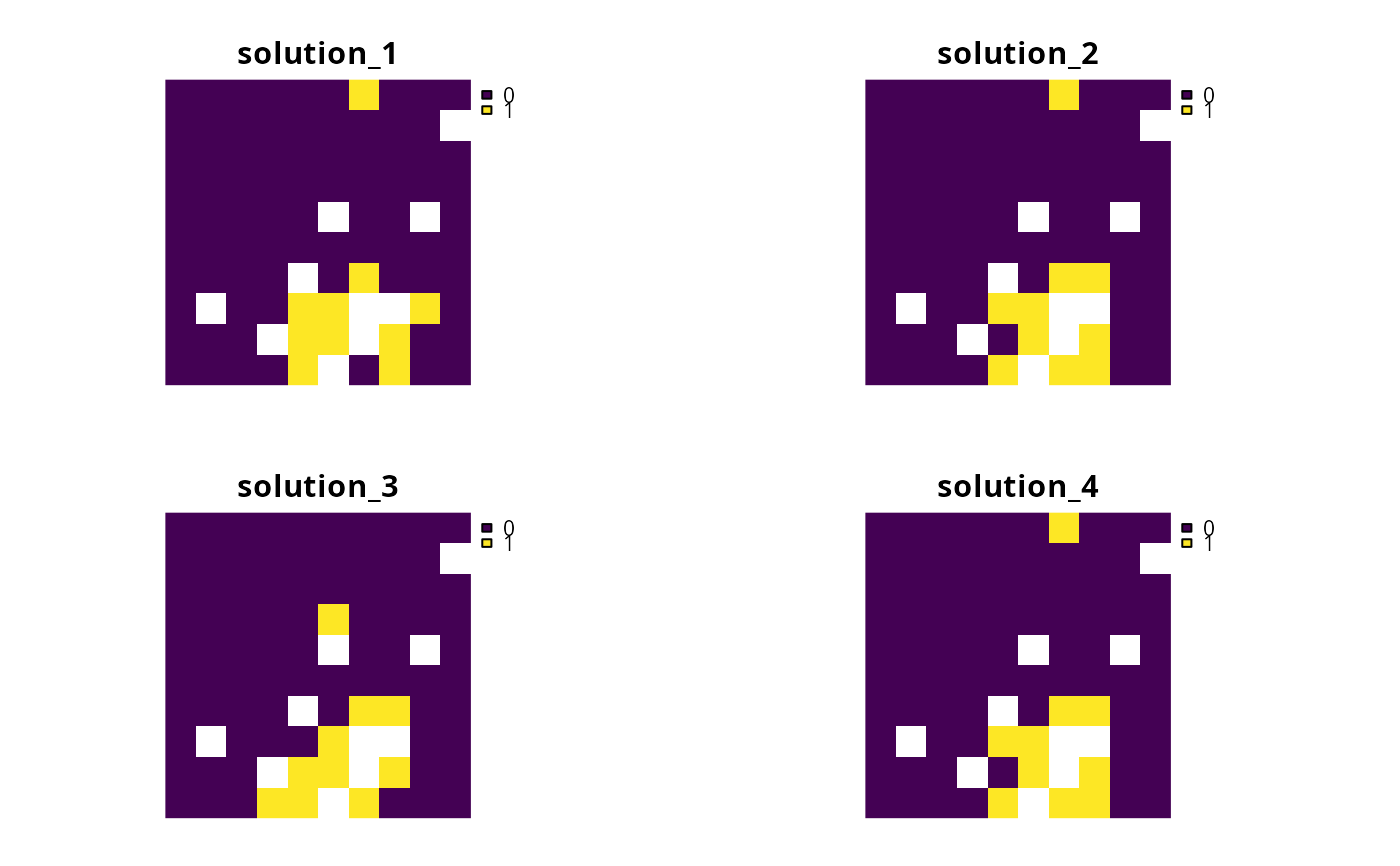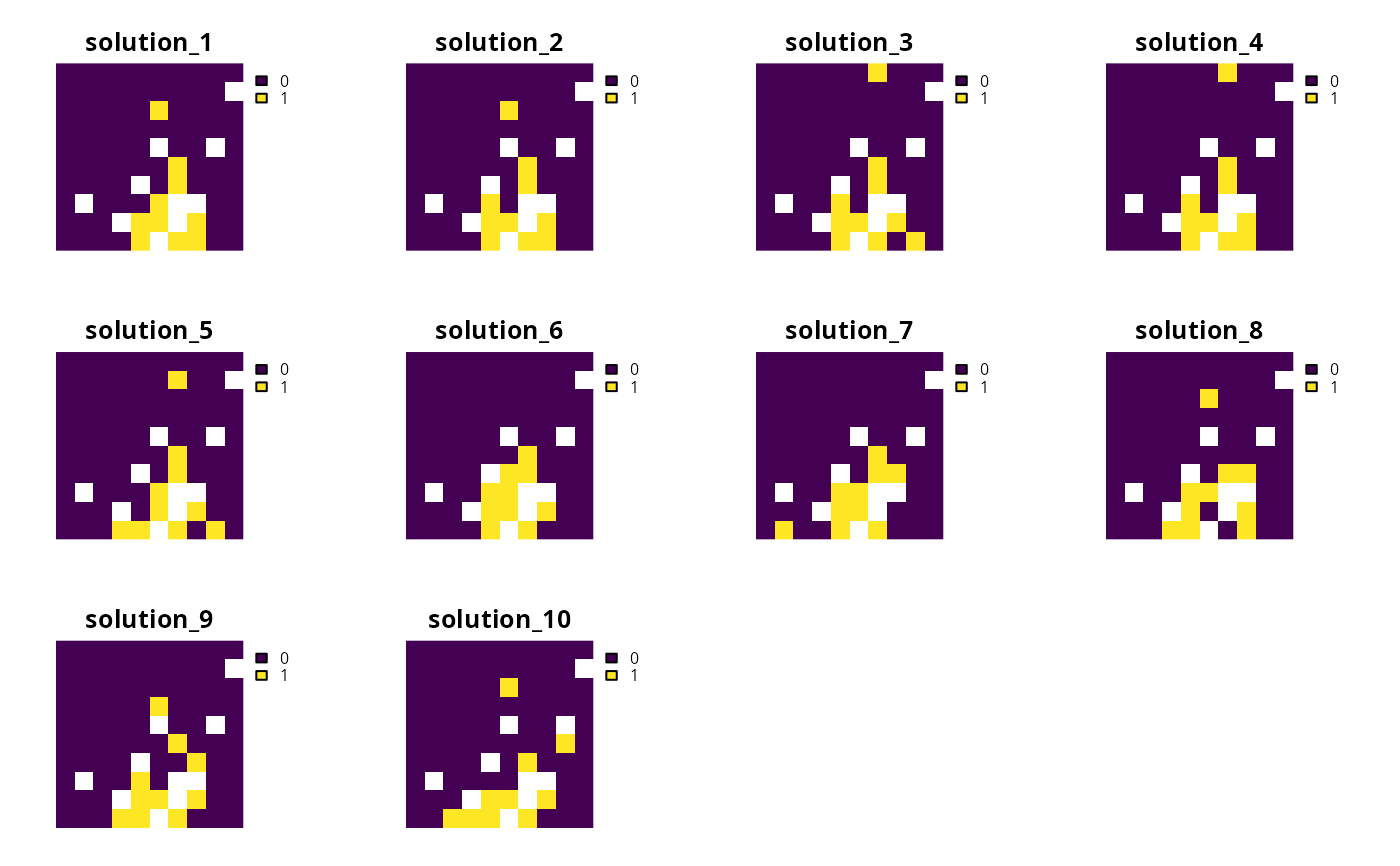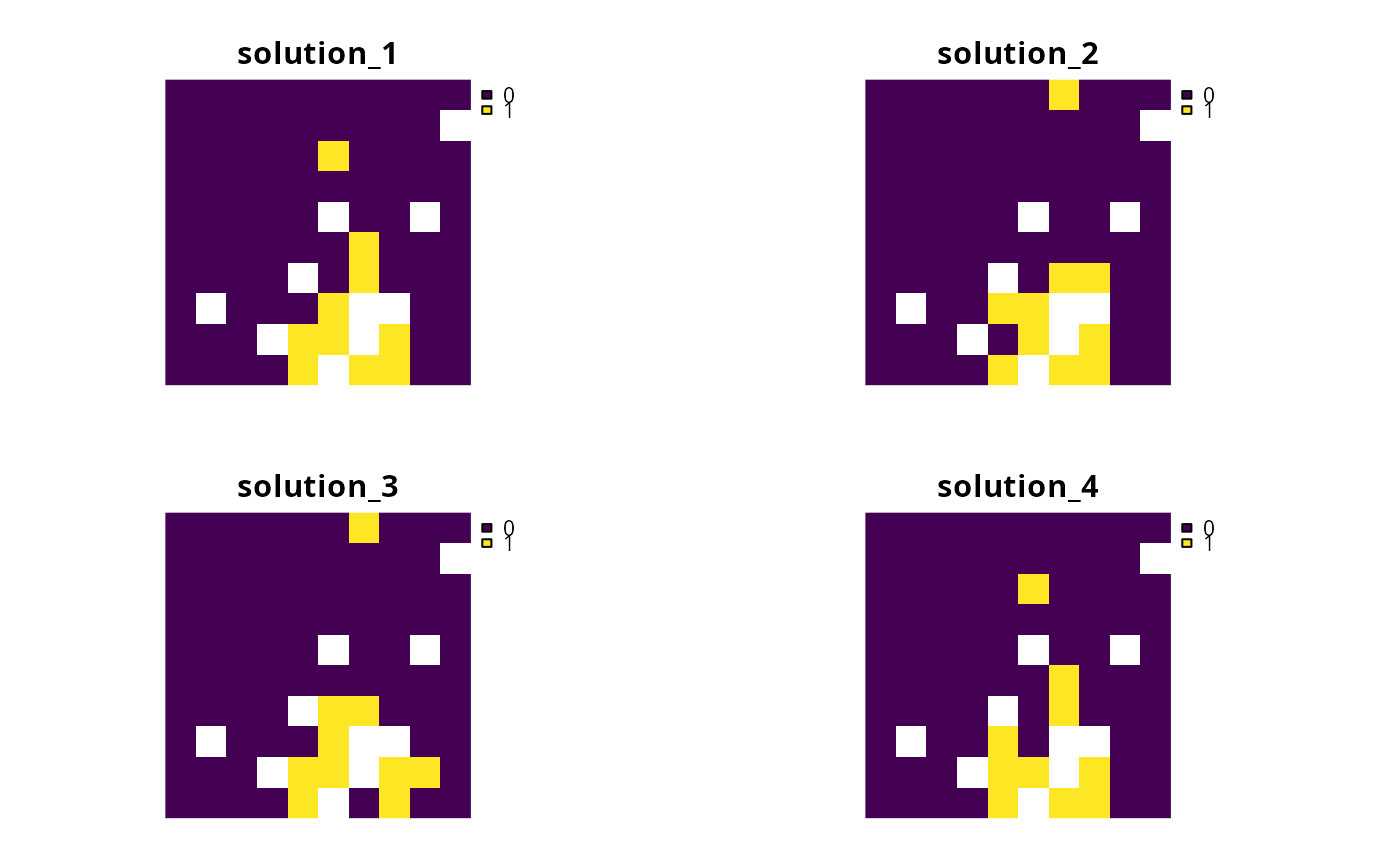Conservation planning exercises rarely have access to all the data needed to identify the truly perfect solution. This is because available data may lack important details (e.g., land acquisition costs may be unavailable), contain errors (e.g., species presence/absence data may have false positives), or key objectives may not be formally incorporated into the prioritization process (e.g., future land use requirements). As such, conservation planners can help decision makers by providing them with a portfolio of solutions to inform their decision.

## Details

The following portfolios can be added to a conservation planning problem(). Note that all methods for generating portfolios return solutions that are within the specified optimality gap.

add_extra_portfolio()

Generate a portfolio of solutions by storing feasible solutions found during the optimization process. This method is useful for quickly obtaining multiple solutions, but does not provide any guarantees on the number of solutions, or the quality of solutions. Note that it requires the Gurobi solver.

add_top_portfolio()

Generate a portfolio of solutions by finding a pre-specified number of solutions that are closest to optimality (i.e., the top solutions). This is useful for examining differences among near-optimal solutions. It can also be used to generate multiple solutions and, in turn, to calculate selection frequencies for small problems. Note that it requires the Gurobi solver.

add_gap_portfolio()

Generate a portfolio of solutions by finding a certain number of solutions that are all within a pre- specified optimality gap. This method is useful for generating multiple solutions that can be used to calculate selection frequencies for moderate and large-sized problems (similar to Marxan). Note that it requires the Gurobi solver.

add_cuts_portfolio()

Generate a portfolio of distinct solutions within a pre-specified optimality gap using Bender's cuts. This is recommended as a replacement for add_top_portfolio() when the Gurobi software is not available.

add_shuffle_portfolio()

Generate a portfolio of solutions by randomly reordering the data prior to attempting to solve the problem. This is recommended as a replacement for add_gap_portfolio() when the Gurobi software is not available.

Other overviews: constraints, decisions, importance, objectives, penalties, solvers, summaries, targets

## Examples

# \dontrun{
sim_pu_raster <- get_sim_pu_raster()
sim_features <- get_sim_features()

# create problem
p <-
problem(sim_pu_raster, sim_features) %>%
add_default_solver(gap = 0.02, verbose = FALSE)

# create problem with cuts portfolio with 4 solutions

# create problem with shuffle portfolio with 4 solutions

# create problem with extra portfolio

# create problem with top portfolio with 4 solutions

# create problem with gap portfolio with 4 solutions within 50% of optimality
p5 <- p %>% add_gap_portfolio(4, 0.5)

# solve problems and create solution portfolios
s <- list(solve(p1), solve(p2), solve(p3), solve(p4), solve(p5))
#> Warning: Portfolio could only find 3 out of 4 solutions.

# plot solutions from cuts portfolio
plot(terra::rast(s[]), axes = FALSE)# plot solutions from shuffle portfolio
plot(terra::rast(s[]), axes = FALSE)# plot solutions from extra portfolio
plot(terra::rast(s[]), axes = FALSE)# plot solutions from top portfolio
plot(terra::rast(s[]), axes = FALSE)# plot solutions from gap portfolio
plot(terra::rast(s[]), axes = FALSE)# }